# Important Questions For Class 10 Maths - Chapter 10 Circles

Important questions for Class 10 Maths Chapter 10 Circles are provided here based on the new pattern of CBSE. Students who are preparing for the board exams can practice these important questions of Circles For Class 10 to score full marks for the questions from this chapter. Students may come across a few of these questions in the board exam. So, they must practice them well and give 100% for the exam preparation.

Chapter 10 Circles has less number of questions, and there is a scope that objective-based questions, including short answer and long answer questions, will occur in the board examination. Students are advised to refer to these important questions for class 10 Maths as a part of their board exam preparation. Along with the important questions, a detailed explanation is also provided. Students can refer to it anytime when they find themselves stuck with the question.

Also, get the MCQs for Class 10 Maths Chapter 10 Circles here.

## Important Questions & Answers For Class 10 Maths Chapter 10 Circles

Important questions with solutions for chapter 10 circles are given here for class 10 students, who are going to give board exams. Practice these questions and increase your chances to score good marks.

Q.1: How many tangents can be drawn from the external point to a circle?

Answer: Two tangents can be drawn from the external point to a circle.

Q.2: Given: A triangle OAB which is an isosceles triangle and AB is tangent to the circle with centre O. Find the measure of ∠OAB.

Answer: The measure of ∠OAB in the given isosceles triangle OAB will be 45 degrees.

Q.3: What should be the angle between the two tangents which are drawn at the end of two radii and are inclined at an angle of 45 degrees?

Answer: The angle between them shall be 135 degrees.

Q.4: Given a right triangle PQR which is right-angled at Q. QR = 12 cm, PQ = 5 cm. The radius of the circle which is inscribed in triangle PQR will be?

Q.5: Define Tangent and Secant.

Answer: A tangent is a line which meets the circle only at one point.

A secant is a line which meets the circle at two points while intersecting it. These two points are always distinct.

Q.6: What is a circle?

Answer: If we collect all the points given on a plane and are at a constant distance, we will get a circle. The constant distance is the radius and the fixed point will be the centre of the circle.

Q.1: From a point Q, the length of the tangent to a circle is 24 cm and the distance of Q from the centre is 25 cm. Find the radius of the circle.

Solution:

First, draw a perpendicular from the centre O of the triangle to a point P on the circle which is touching the tangent. This line will be perpendicular to the tangent of the circle.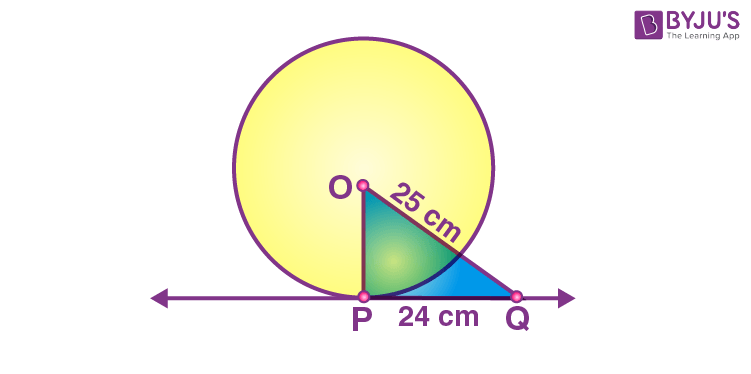So, OP is perpendicular to PQ i.e. OP ⊥ PQ

From the above figure, it is also seen that △OPQ is a right-angled triangle.

It is given that

OQ = 25 cm and PQ = 24 cm

By using Pythagorean theorem in △OPQ,

OQ2 = OP2 + PQ2

=> (25)2 = OP2 + (24)2

=> OP2 = 625 – 576

=> OP2 = 49

=> OP = 7 cm

Therefore, the radius of the given circle is 7 cm.

Q. 2: Prove that the tangents drawn at the ends of a diameter of a circle are parallel.

Solution:

First, draw a circle and connect two points A and B such that AB becomes the diameter of the circle. Now, draw two tangents PQ and RS at points A and B respectively.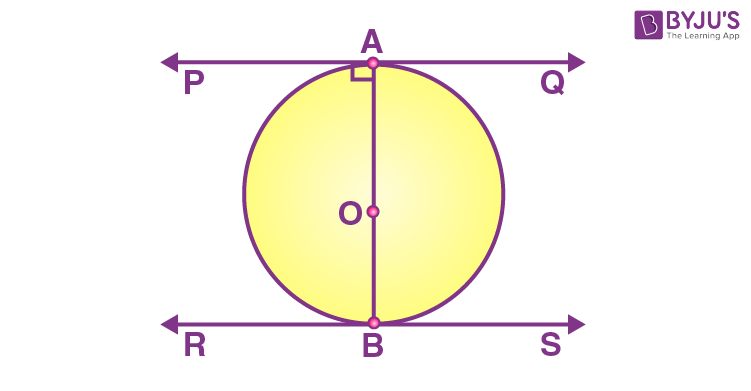Now, both radii i.e. AO and OB are perpendicular to the tangents.

So, OB is perpendicular to RS and OA perpendicular to PQ

So, ∠OAP = ∠OAQ = ∠OBR = ∠OBS = 90°

From the above figure, angles OBR and OAQ are alternate interior angles.

Also, ∠OBR = ∠OAQ and ∠OBS = ∠OAP {since they are also alternate interior angles}

So, it can be said that line PQ and the line RS will be parallel to each other.

Hence Proved.

Q. 3: A quadrilateral ABCD is drawn to circumscribe a circle as shown in the figure. Prove that AB + CD = AD + BC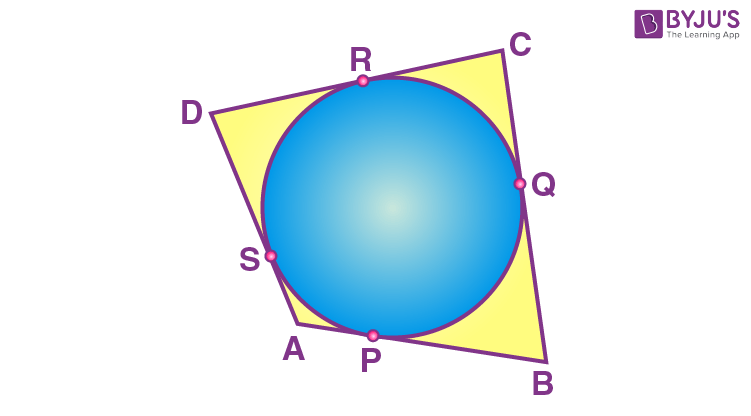Solution:

From this figure,

(i) DR = DS

(ii) BP = BQ

(iii) AP = AS

(iv) CR = CQ

Since they are tangents on the circle from points D, B, A, and C respectively.

Now, adding the LHS and RHS of the above equations we get,

DR + BP + AP+ CR = DS + BQ + AS + CQ

By rearranging them we get,

(DR + CR) + (BP + AP) = (CQ + BQ) + (DS + AS)

By simplifying,

AD + BC = CD + AB

Q. 4: Two concentric circles are of radii 5 cm and 3 cm. Find the length of the chord of the larger circle which touches the smaller circle.

Solution:

Draw two concentric circles with the centre O. Now, draw a chord AB in the larger circle which touches the smaller circle at a point P as shown in the figure below.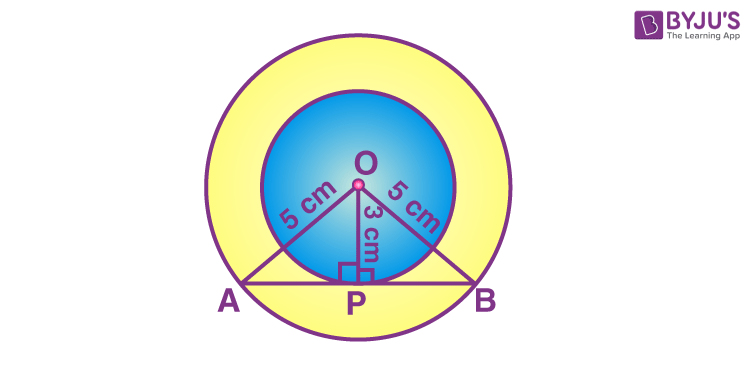From the above diagram, AB is tangent to the smaller circle to point P.

∴ OP ⊥ AB

Using Pythagoras theorem in triangle OPA,

OA2 = AP2 + OP2

=> 52 = AP2 + 32

=> AP2 = 25 – 9 = 16

=> AP = 4

OP ⊥ AB

Since the perpendicular from the centre of the circle bisects the chord, AP will be equal to PB

So, AB = 2AP = 2 × 4 = 8 cm

Hence, the length of the chord of the larger circle is 8 cm.

Q. 5: Let s denote the semi-perimeter of a triangle ABC in which BC = a, CA = b, AB = c. If a circle touches the sides BC, CA, AB at D, E, F, respectively, prove that BD = s – b.

Solution:

According to the question,

A triangle ABC with BC = a , CA = b and AB = c . Also, a circle is inscribed which touches the sides BC, CA and AB at D, E and F respectively and s is semi perimeter of the triangle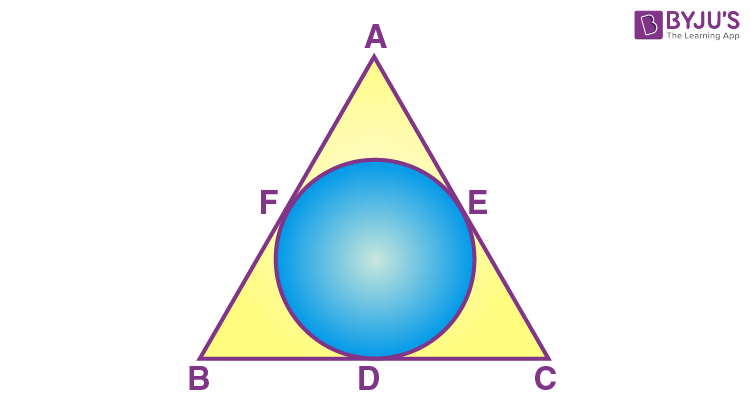To Prove: BD = s – b

Proof:

According to the question,

We have,

Semi Perimeter = s

Perimeter = 2s

2s = AB + BC + AC…. 

As we know,

Tangents drawn from an external point to a circle are equal

So we have

AF = AE…  [Tangents from point A]

BF = BD …. [Tangents From point B]

CD = CE….  [Tangents From point C]

AF + BF + CD = AE + BD + CE

AB + CD = AC + BD

AB + CD + BD = AC + BD + BD

AB + BC – AC = 2BD

AB + BC + AC – AC – AC = 2BD

2s – 2AC = 2BD [From (1)]

2BD = 2s – 2b [as AC = b]

BD = s – b

Hence proved.

Q.6: In the figure, two tangents TP and TQ are drawn to a circle with centre O from an external point T, prove that ∠PTQ = 2OPQ.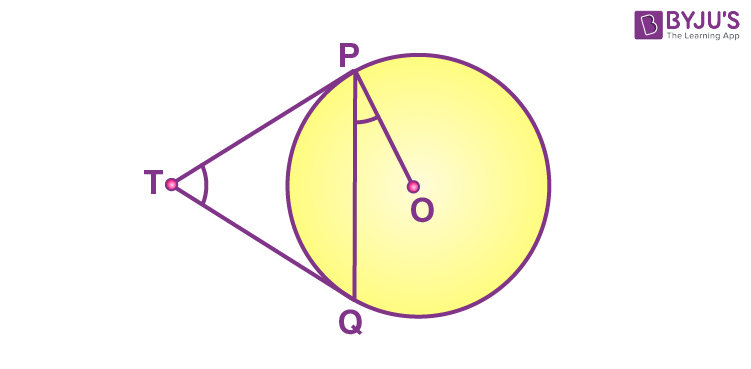Solution:

Given that two tangents TP and TQ are drawn to a circle with centre O from an external point T

Let ∠PTQ = θ.

Now, by using the theorem “the lengths of tangents drawn from an external point to a circle are equal”, we can say TP = TQ. So, TPQ is an isosceles triangle.

Thus,

∠TPQ = ∠TQP = ½ (180°− θ ) = 90° – (½) θ

By using the theorem, “the tangent at any point of a circle is perpendicular to the radius through the point of contact”, we can say ∠OPT = 90°

Therefore,

∠OPQ = ∠OPT – ∠TPQ = 90° – [90° – (½) θ]

∠OPQ = (½)θ

∠OPQ = (½) ∠PTQ

⇒ ∠PTQ = 2 ∠OPQ.

Hence proved.

Q.7: Prove that the lengths of tangents drawn from an external point to a circle are equal.

Solution:

Consider a circle with the centre “O” and P is the point that lies outside the circle. Hence, the two tangents formed are PQ and PR.

We need to prove: PQ = PR.

To prove the tangent PQ is equal to PR, join OP, OQ and OR. Hence, ∠OQP and ∠ORP are the right angles.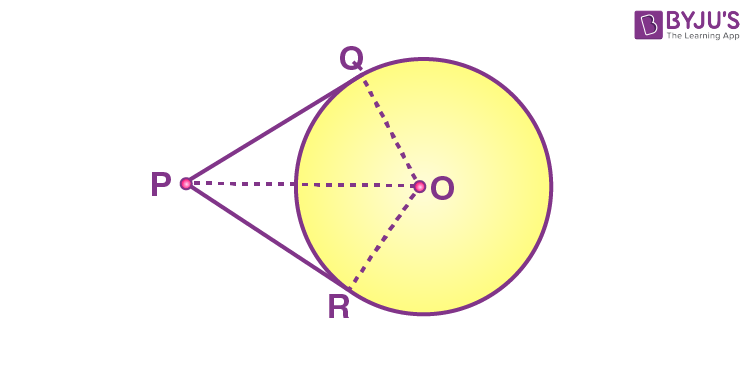OP = OP (Common side)

By using the RHS rule, we can say, ∆ OQP ≅ ∆ ORP.

Thus, by using the CPCT rule, the tangent PQ = PR.

Hence proved.

Q.8: In the figure, from an external point P, two tangents PT and PS are drawn to a circle with centre O and radius r. If OP = 2r, show that ∠OTS = ∠OST = 30°.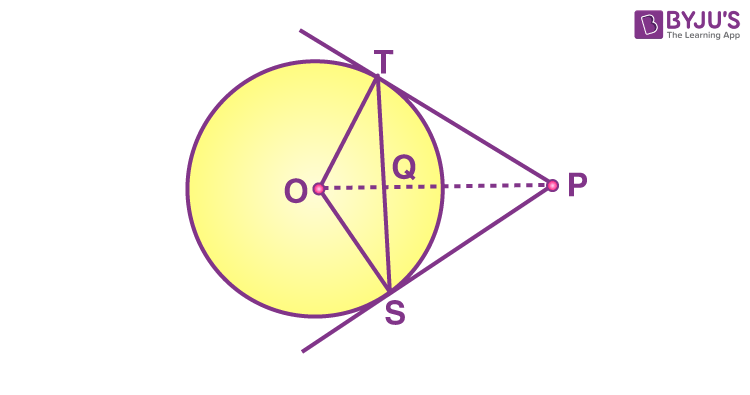Solution:

Given that from an external point P, Two tangents PT and PS are drawn to a circle with center O and radius r and OP = 2r

OS = OT {radii of same circle}

∠OTS = ∠OST {angles opposite to equal sides are equal} ….(i)

A tangent drawn at a point on a circle is perpendicular to the radius through point of contact.

OT ⏊ TP and OS ⏊ SP

∠OSP = 90°

∠OST + ∠PST = 90°

∠PST = 90° – ∠OST….(ii)

In triangle PTS

PT = PS {tangents drawn from an external point to a circle are equal}

∠PST = ∠PTS = 90° – ∠OST {from (ii)}

In △PTS

∠PTS + ∠PST + ∠SPT = 180° {angle sum property of a triangle}

90° – ∠OST + 90° – ∠OST + ∠SPT = 180°

∠SPT = 2∠OST….(iii)

In △OTP, OT⏊TP

sin(∠OPT) = OT/OP = r/2r = 1/2

sin(∠OPT) = sin 30°

∠OPT = 30°….(iv)

Similarly,

In △OSP,

∠OPS = 30°….(v)

∠OPT + ∠OPS = 30° + 30°

∠SPT = 60°

Now substituting this value in (iii),

∠SPT = 2∠OST

60° = 2∠OST

∠OST = 30°….(vi)

From (i) and (vi),

∠OST = ∠OTS = 30°

Q.9: In the figure, two tangents RQ and RP are drawn from an external point R to the circle with centre O. If ∠PRQ = 120°, then prove that OR = PR + RQ.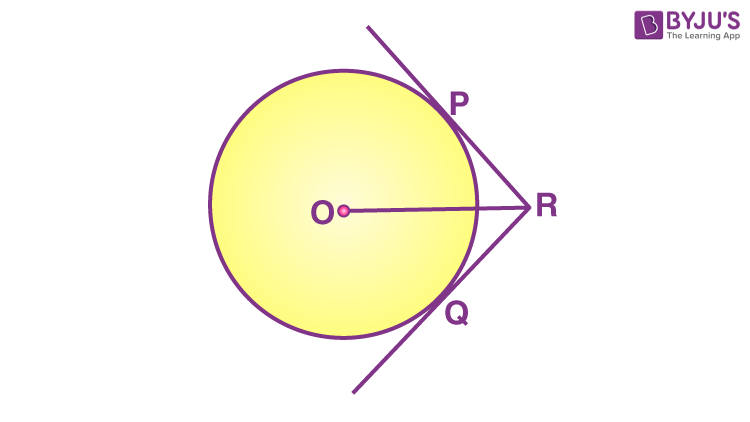Solution:

Given, two tangents RQ and RP are drawn from an external point R to the circle with centre O. ∠PRQ = 120°

Join OP, OQ and OR.

∠PRQ = ∠QRO = 120°/2 = 60°

RQ and RP are the tangent to the circle.

OQ ⊥ QR and OP ⊥ PR

Form right ∆OPR,

∠POR = 180° – (90° + 60°) = 30°

and ∠QOR = 30°

cos a = PR/OR (suppose ‘a’ be the angle)

cos 60° =PR/OR

1/2 = PR/OR

OR = 2 PR

Again from right ∆OQR,

OR = 2 QR

From both the results, we have

2 PR + 2 QR = 2OR

or OR = PR + RQ

Hence Proved.

Q.10: Prove that the tangent drawn at the mid-point of an arc of a circle is parallel to the chord joining the endpoints of the arc.

Solution:

Let mid-point of an arc AMB be M and TMT’ be the tangent to the circle.

Now, join AB, AM and MB.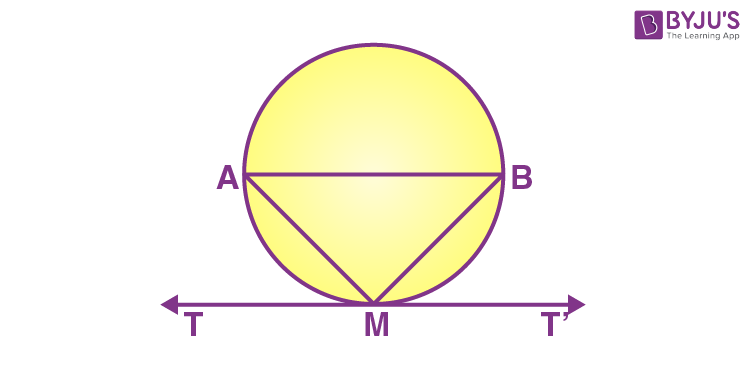Since, arc AM = arc MB

⇒ Chord AM = Chord MB

In ∆AMB, AM = MB

⇒ ∠MAB = ∠MBA….(i) {angles corresponding to the equal sides are equal}

Since, TMT’ is a tangent line.

∠AMT = ∠MBA {angles in alternate segment are equal}

Thus, ∠AMT = ∠MAB {from (i)}

But ∠AMT and ∠MAB are alternate angles, which is possible only when AB || TMT’

Therefore, the tangent drawn at the mid-point of an arc of a circle is parallel to the chord joining the end points of the arc.

Hence proved.

### Practice Questions For Class 10 Maths Chapter 10 Circles

1. How many tangents can a circle have?
2. Prove that the parallelogram circumscribing a circle is a rhombus.
3. In a right triangle ABC in which ∠B = 90°, a circle is drawn with AB as diameter intersecting the hypotenuse AC and P. Prove that the tangent to the circle at P bisects BC.
4. If an isosceles triangle ABC, in which AB = AC = 6 cm, is inscribed in a circle of radius 9 cm, find the area of the triangle.
5. In the figure, PQ and RS are two parallel tangents to a circle with centre O and another tangent AB with point of contact C intersecting PQ at A and RS at B. Prove that ∠AOB = 90°.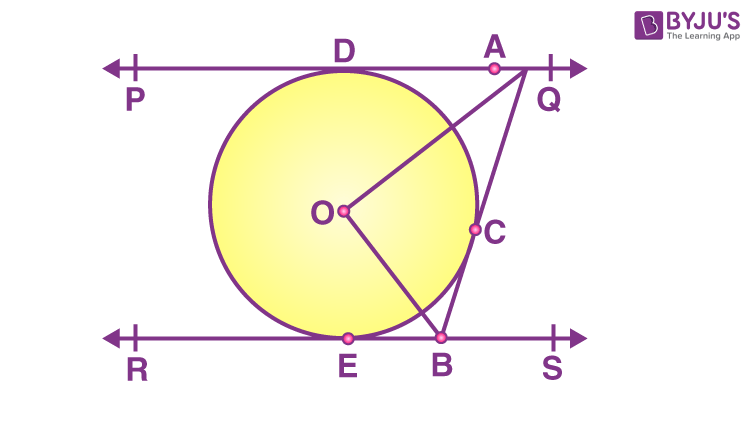6. If the angle between two tangents drawn from an external point P to a circle of radius a and centre O are 60°, then find the length of OP.
7. In the figure, PQ is a tangent at a point C to a circle with centre O. If AB is a diameter and ∠CAB = 30°, find ∠PCA.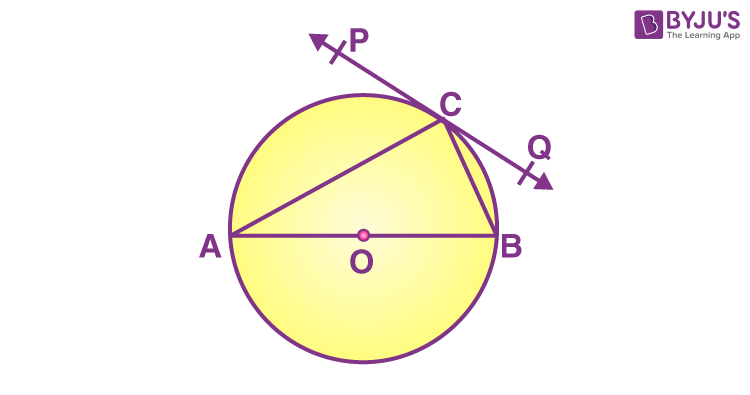8. In the figure, PQ is a chord of a circle with centre O and PT is a tangent. If ∠QPT = 60°, ∠PRQ.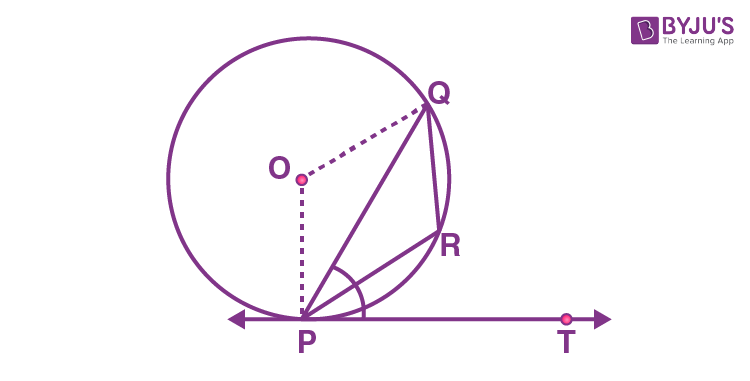9. Prove that the line segment joining the point of contact of two parallel tangents of a circle passes through its centre.
10. If from an external point P of a circle with centre O, two tangents PQ and PR are drawn such that ∠QPR = 120°, prove that 2PQ = PO.

#### 1 Comment

1. the chat section is so usefull and clears all the doubts in some seconds , thanks for providing such good services